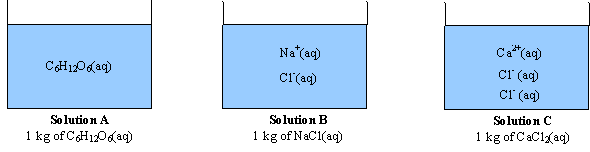# Define Van’t Hoff’s factor. What for it used?

Wiki- The van ‘t Hoff factor is the ratio between the actual concentration of particles produced when the substance is dissolved, and the concentration of a substance as calculated from its mass. For most non-electrolytes dissolved in water, the van’ t Hoff factor is essentially 1. For most ionic compounds dissolved in water, the van ‘t Hoff factor is equal
to the number of discrete ions in a formula unit of the substance. This is true for ideal solutions only. Sometime ion pairing occurs in solution. At a given instant a small percentage of the ions are paired and count as a single particle. Ion pairing occurs to some extent in all electrolyte solutions. This causes deviation from the van ‘t Hoff factor. The deviation for the van ‘t Hoff factor tends to be greatest where the ions have multiple charges.i=van’t Hoff= the number of particles a formula breaks up into

C6H12O6(s) ==>C6H12O6(aq)    (1 mole of particles)       C6H12O=1

NaCl(s) ==> Na+(aq) + Cl-(aq)    (2 moles of particles)      NaCl=2

CaCl2(s) ==> Ca2+(aq) + 2Cl-(aq)   (3 moles of particles)    CaCl2=3